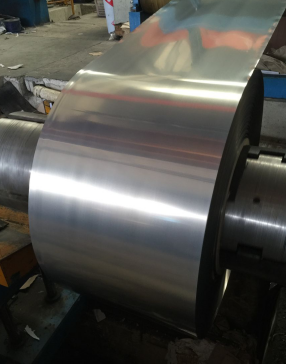# 304不锈钢卷板，304抛光不锈钢卷带

2 件

(发货期限：自买家付款之日起 3 天内发货)

• 详细说明
• 规格参数
• 联系方式

200系列：201/202

400系列：value="409" hasspace="False" negative="False" numbertype="1" tcsc="0" w:st="on">409L/410/430/420/441/443/value="436" hasspace="False" negative="False" numbertype="1" tcsc="0" w:st="on">436L/439

300系列：301/304/value="304" hasspace="False" negative="False" numbertype="1" tcsc="0" w:st="on">304L/309S/310S/316/value="316" hasspace="False" negative="False" numbertype="1" tcsc="0" w:st="on">316L/value="317" hasspace="False" negative="False" numbertype="1" tcsc="0" w:st="on">317L/321• 所在地区：广东-佛山市